# Chemical Formulas The Basics Subscripts in Chemical Formulas

• Slides: 18Chemical Formulas: The Basics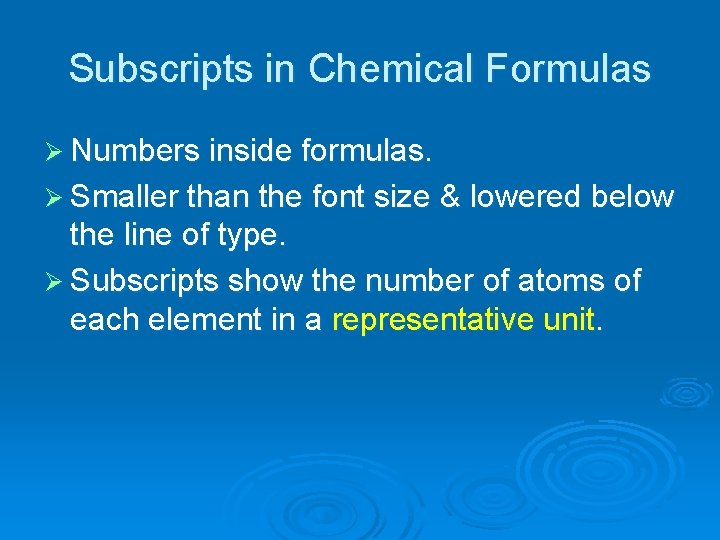Subscripts in Chemical Formulas Ø Numbers inside formulas. Ø Smaller than the font size & lowered below the line of type. Ø Subscripts show the number of atoms of each element in a representative unit.Rules for Subscripts in Formulas Ø No subscript – it’s understood to be 1. Ø Subscripts refer to the element immediately preceding them. Remember – every element symbol starts with an upper case letter. Ø Subscripts after parentheses refer to everything inside ( ).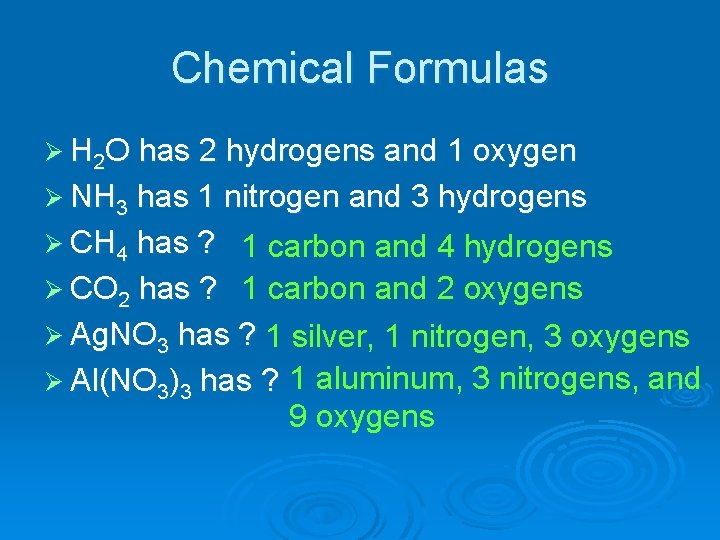Chemical Formulas Ø H 2 O has 2 hydrogens and 1 oxygen Ø NH 3 has 1 nitrogen and 3 hydrogens Ø CH 4 has ? 1 carbon and 4 hydrogens Ø CO 2 has ? 1 carbon and 2 oxygens Ø Ag. NO 3 has ? 1 silver, 1 nitrogen, 3 oxygens Ø Al(NO 3)3 has ? 1 aluminum, 3 nitrogens, and 9 oxygens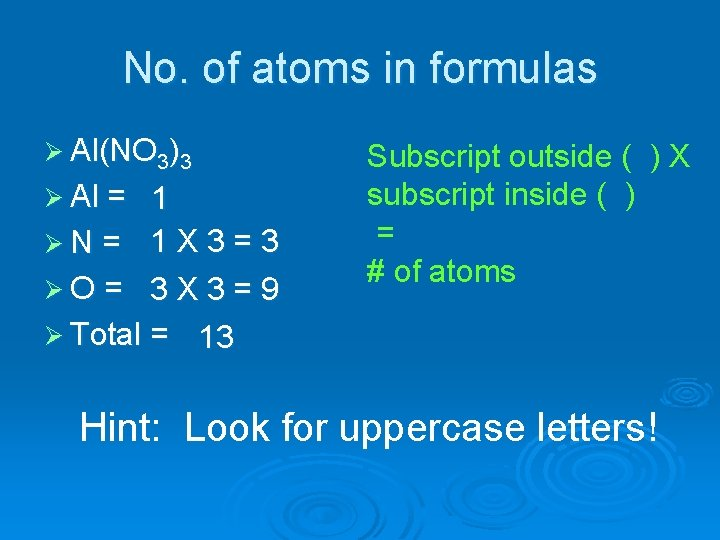No. of atoms in formulas Ø Al(NO 3)3 Ø Al = 1 ØN = 1 X 3 = 3 ØO = 3 X 3 = 9 Ø Total = 13 Subscript outside ( ) X subscript inside ( ) = # of atoms Hint: Look for uppercase letters!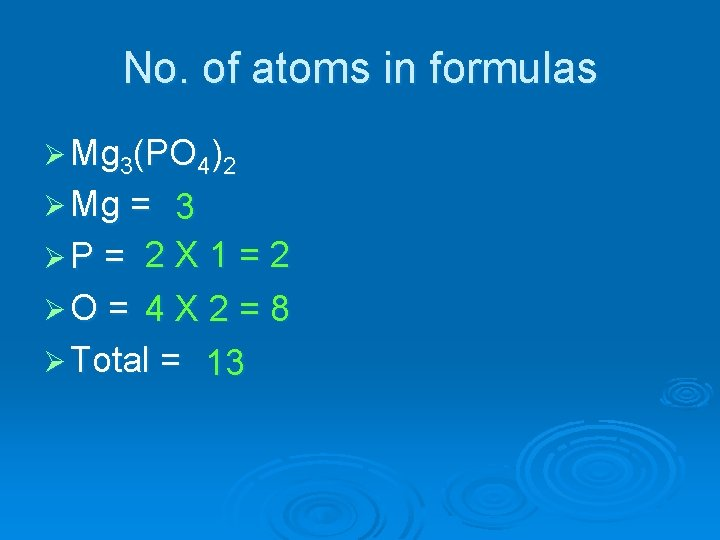No. of atoms in formulas Ø Mg 3(PO 4)2 Ø Mg = 3 ØP = 2 X 1 = 2 ØO = 4 X 2 = 8 Ø Total = 13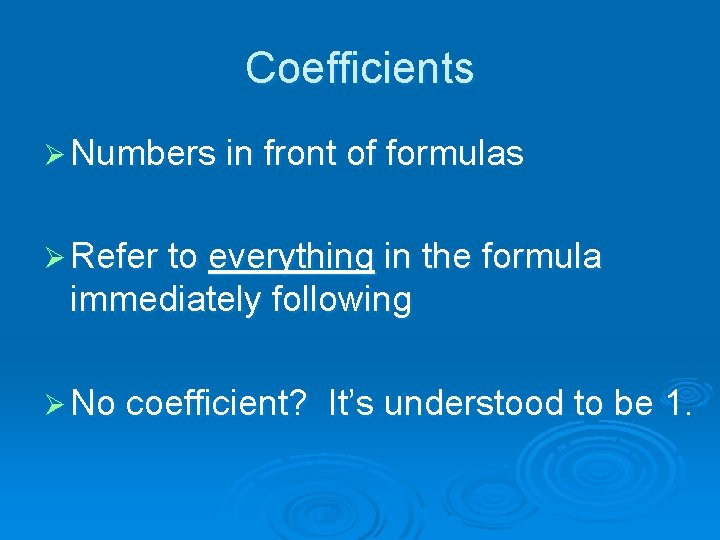Coefficients Ø Numbers in front of formulas Ø Refer to everything in the formula immediately following Ø No coefficient? It’s understood to be 1.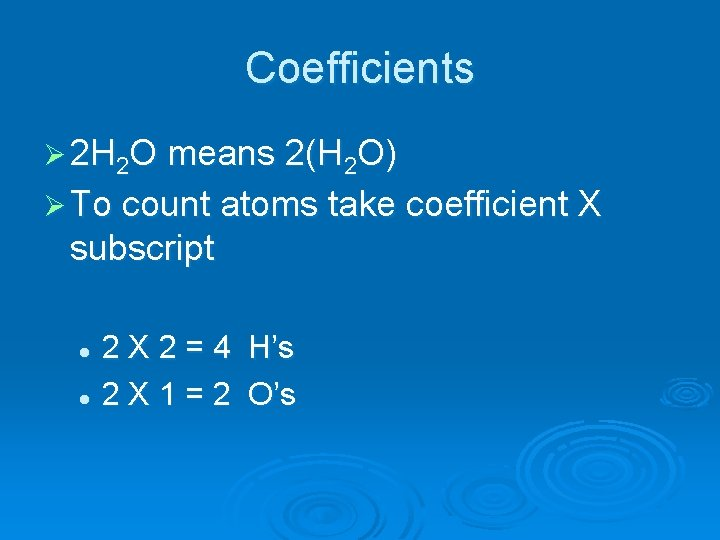Coefficients Ø 2 H 2 O means 2(H 2 O) Ø To count atoms take coefficient X subscript 2 X 2 = 4 H’s l 2 X 1 = 2 O’s lHydrates Ø A group of salts that have water molecules stuffed in the empty spaces Ø Formulas are distinctive Ø Ex: Cu. SO 4 5 H 2 O l l means “is associated with” or PLUS Not a true chemical bond, but the structure is definite so the number of empty spaces is definite and the number of water molecules is definiteCu. SO 4 5 H 2 O Ø Count up the atoms! 1 X Cu 1 XS 4 XO 10 X H 5 XO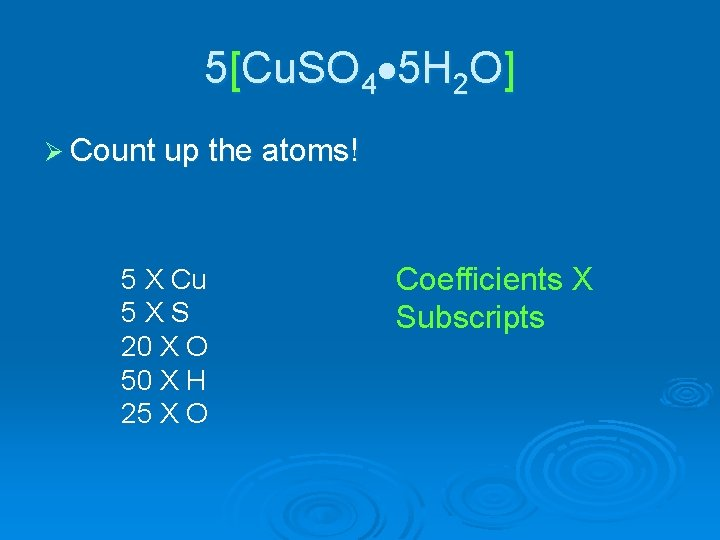5[Cu. SO 4 5 H 2 O] Ø Count up the atoms! 5 X Cu 5 XS 20 X O 50 X H 25 X O Coefficients X Subscripts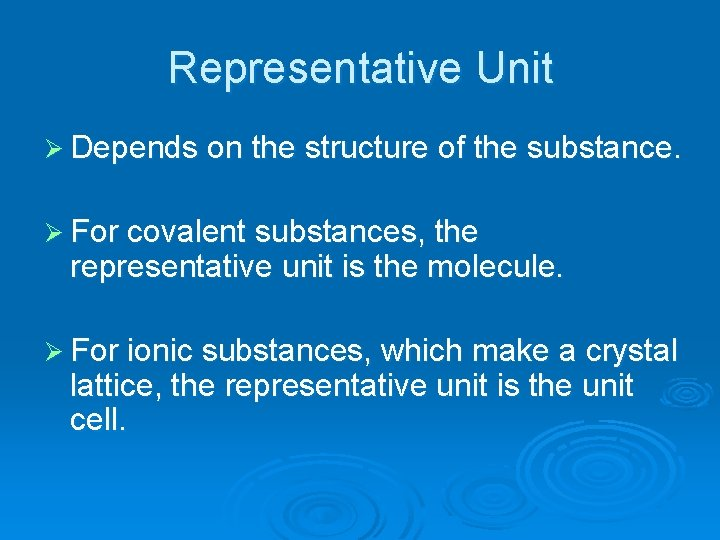Representative Unit Ø Depends on the structure of the substance. Ø For covalent substances, the representative unit is the molecule. Ø For ionic substances, which make a crystal lattice, the representative unit is the unit cell.Covalently bonded substances make molecules. If you have more raw materials, you make more molecules.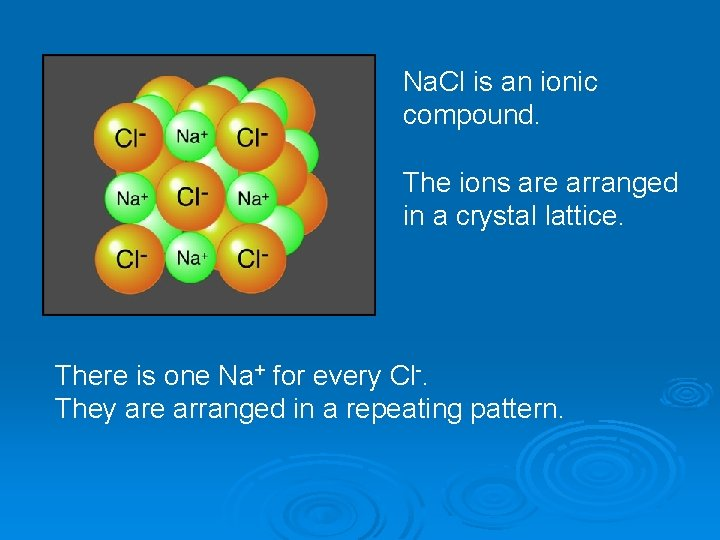Na. Cl is an ionic compound. The ions are arranged in a crystal lattice. There is one Na+ for every Cl-. They are arranged in a repeating pattern.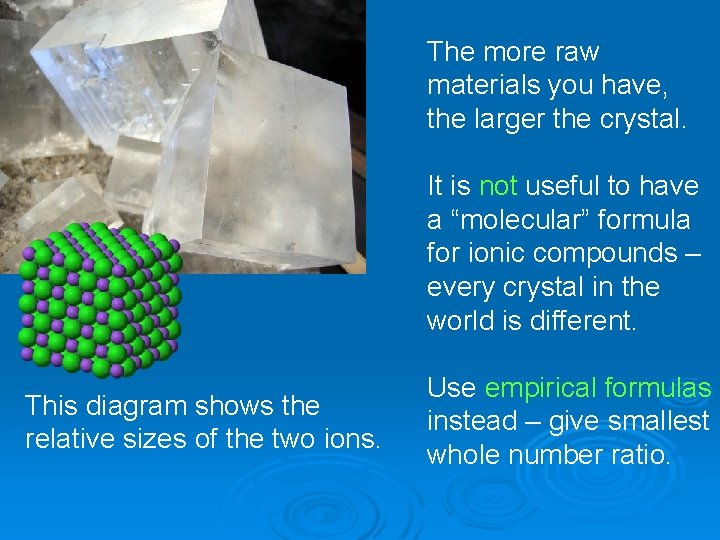The more raw materials you have, the larger the crystal. It is not useful to have a “molecular” formula for ionic compounds – every crystal in the world is different. This diagram shows the relative sizes of the two ions. Use empirical formulas instead – give smallest whole number ratio.When all the ions are in the correct location, you can get a large “single crystal. ”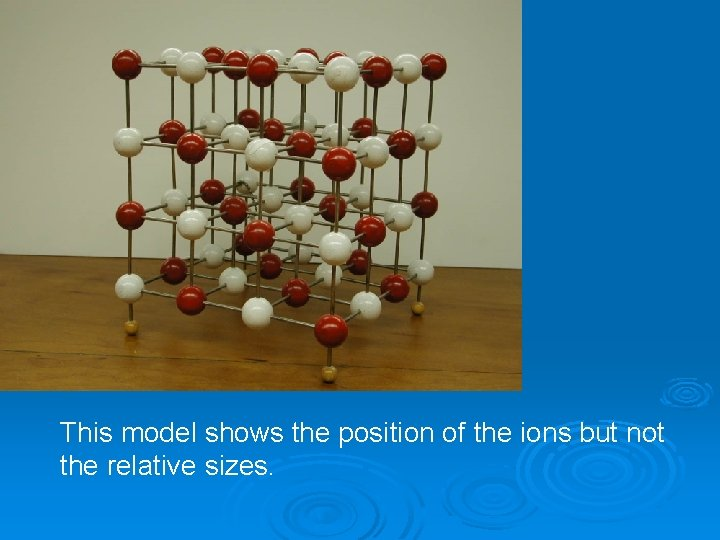This model shows the position of the ions but not the relative sizes.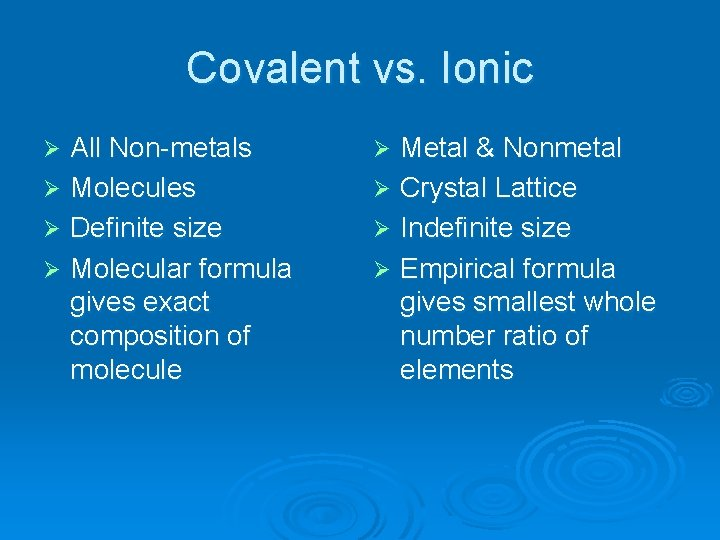Covalent vs. Ionic All Non-metals Ø Molecules Ø Definite size Ø Molecular formula gives exact composition of molecule Ø Metal & Nonmetal Ø Crystal Lattice Ø Indefinite size Ø Empirical formula gives smallest whole number ratio of elements Ø